# Pseudoprime

A pseudoprime is a composite number that has certain properties in common with prime numbers.

##  Introduction

To find out if a given number is a prime number, one can test it for properties that all prime numbers share. One property of a prime number is that it is only divisible by one and itself. This is a defining property: it holds for all primes and no other numbers.

However, other properties hold for all primes and also some other numbers. For instance, every prime number greater than 3 has the form$6n - 1\$ or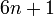$6n + 1\$ (with n an integer), but there are also composite numbers of this form: 25, 35, 49, 55, 65, 77, 85, 91, … . So, we can say that 25, 35, 49, 55, 65, 77, 85, 91, … are pseudoprimes with respect to the property of being of the form$6n - 1\$ or$6n + 1\$. There exist better properties, which lead to special pseudoprimes, as outlined below.

##  Different kinds of pseudoprimes

Property kind of pseudoprime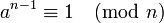$a^{n-1} \equiv 1 \pmod{n}$ Fermat pseudoprime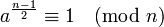$a^{\frac{n-1}{2}} \equiv 1 \pmod{n}$ Euler pseudoprime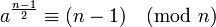$a^{\frac{n-1}{2}} \equiv (n-1) \pmod{n}$$a^d \equiv 1 \pmod{n}$ strong pseudoprime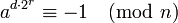$a^{d\cdot2^r} \equiv -1 \pmod{n}$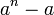$a^n - a\$ is divisible by$n\$ Carmichael number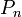$P_n\$ is divisible by$n\$ Perrin pseudoprime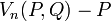$V_n(P,Q) - P\$ is divisible by$n\$

##  Table of smallest Pseudoprimes

smallest Pseudoprimes
Number Kind of Pseudoprime Bases
15 Fermat pseudoprime 4, 11
21 Euler pseudoprime 8, 13
49 strong pseudoprime 18, 19, 30, 31
561 Carmichael number
1729 absolute Euler pseudoprimeSome content on this page may previously have appeared on Citizendium.